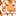# QlikView App Dev

Discussion Board for collaboration related to QlikView App Development.

Announcements
cancel
Showing results for
Did you mean:Creator

## Calculate number of months occurring in current year only

Hi,

I have 2 date columns

Start Date and End Date

ID   Start date    End date

1    1-Nov-16     31-Jul-17

2    1-Aug-17     31-Jul-18

Here I have to calculate number of months occurring in 2017 only.Output should be as below

ID   Start date    End date   NoOfMonths

1    1-Nov-16     31-Jul-17     7                          (b/w Nov-2016 and Jul 2017  total  7 months are in 2017)

2    1-Aug-17     31-Jul-18     5                          (b/w Aug-2017 and Jul 2018  total   5 months are in 2017)

How can I put this in script ?

Thank you very much

1 Solution

Accepted SolutionsMVP

May be this:

Table:

If([Start date] >= MakeDate(Year(Today())),

If([End date] < MakeDate(Year(Today()) + 1), Month([End date]) - Month([Start date]), 12 - Month([Start date]) + 1),

If([End date] < MakeDate(Year(Today()) + 1), Num(Month([End date])), 12)) as NoOfMonths;

ID,  Start date,    End date

1,    1-Nov-16,    31-Jul-17

2,    1-Aug-17,    31-Jul-18

];

5 RepliesMVP

May be like this

Num(Month([End date])) as NoOfMonthsMaster

=((year([End date])*12)+month([End date])) - (((year([Start date])*12)+month([Start date])))

This basically creates a numeric 'period' for each date and takes one from the other

whoops didn't notice the 'only in 2017 bit'

=

(if([End date] > yearend(Today()), (((year(yearend(Today()))*12)+month(yearendt(Today())))),(((year([End date])*12)+month([End date]))))

-

(if([Start date] < yearstart(Today()), (((year(yearstart(Today()))*12)+month(yearstart(Today())))),(((year([Start date])*12)+month([Start date]))))MVP

May be this:

Table:

If([Start date] >= MakeDate(Year(Today())),

If([End date] < MakeDate(Year(Today()) + 1), Month([End date]) - Month([Start date]), 12 - Month([Start date]) + 1),

If([End date] < MakeDate(Year(Today()) + 1), Num(Month([End date])), 12)) as NoOfMonths;

ID,  Start date,    End date

1,    1-Nov-16,    31-Jul-17

2,    1-Aug-17,    31-Jul-18

];Creator
Author

Thank you Sunny.Awesomeness...You are my heroChampion III

or just this

*,

if(Year(Startdate)-Year(Enddate)=0,Month(Enddate)-Month(Startdate),if(Year(Startdate)=Year(Today()),12-Month(Startdate),Month(Enddate)-1)+1) as NoOfMonth;

LOAD ID,date(Date#(Startdate,'DD-MMM-YY'),'DD/MM/YY') as Startdate,date(Date#(Enddate,'DD-MMM-YY'),'DD/MM/YY') as Enddate Inline [

ID,Startdate,Enddate

1,1-Nov-16,31-Jul-17

2,1-Aug-17,31-Jul-18

];

Vineeth Pujari
If a post helps to resolve your issue, please accept it as a Solution.